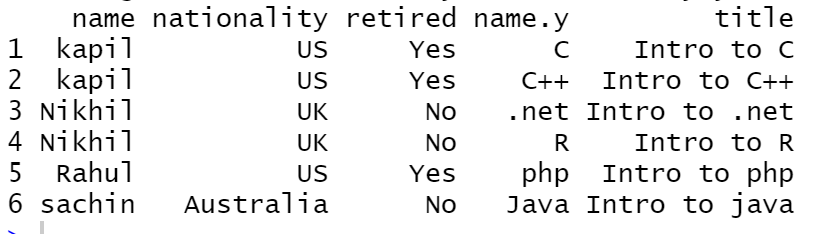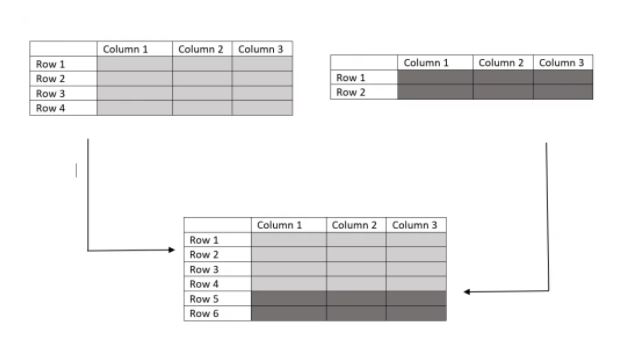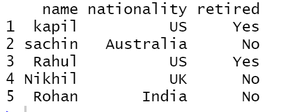Related Articles

# How To Merge Two DataFrames in R ?

• Last Updated : 26 Mar, 2021

In this article, We are going to see how to merge two R dataFrames. Merging of Data frames in R can be done in two ways.

• Merging columns
• Merging rows

### Merging columns

In this way, we merge the database horizontally. We use the merge function to merge two frames by one or more common key variables(i.e., an inner join).

`dataframe_AB = merge(dataframe_A, dataframe_B, by="ID")`

Below is the implementation:

## R

 `# merging two datasets``authors <- ``data.frame``(``  ``name = ``c``(``"kapil"``, ``"sachin"``, ``"Rahul"``,``"Nikhil"``,``"Rohan"``),``  ``nationality = ``c``(``"US"``,``"Australia"``,``"US"``,``"UK"``,``"India"``),``  ``retired = ``c``(``"Yes"``,``"No"``,``"Yes"``,``"No"``,``"No"``))`` ` `books <-``data.frame``( ``  ``name = ``c``(``"C"``, ``"C++"``,``"Java"``,``"php"``,``".net"``,``"R"``),``  ``title = ``c``(``"Intro to C"``,``"Intro to C++"``, ``            ``"Intro to java"``, ``"Intro to php"``, ``            ``"Intro to .net"``, ``"Intro to R"``),``  ``author = ``c``(``"kapil"``, ``"kapil"``,``"sachin"``, ``"Rahul"``,``             ``"Nikhil"``,``"Nikhil"``))``   ` `  ``merge``(authors, books, by.x = ``"name"``, by.y = ``"author"``)`

Output:### Merging Rows

In this way, we merge the data frames vertically and use the rbind() function. rbind stands for row binding. The two data frames must have the same variables but need not be in the same order.

Note: If dataframe_A has variables that dataframe_B doesn’t have, either Delete the extra variables in dataframe_A or create the additional variables in dataframe_B and set them to NA.

As we can see from the below diagram, it combines rows of two dataframes.Below is the implementation:

## R

 `# merging two datasets``authors_A <- ``data.frame``(``  ``name = ``c``(``"kapil"``, ``"sachin"``, ``"Rahul"``),``  ``nationality = ``c``(``"US"``, ``"Australia"``, ``"US"``),``  ``retired = ``c``(``"Yes"``, ``"No"``, ``"Yes"``))`` ` `authors_B <- ``data.frame``(``  ``name = ``c``(``"Nikhil"``, ``"Rohan"``),``  ``nationality = ``c``(``"UK"``, ``"India"``),``  ``retired = ``c``(``"No"``, ``"No"``))`` ` `rbind``(authors_A, authors_B)`

Output:My Personal Notes arrow_drop_up